Shortcuts

# Reinforcement Learning¶

This module is a collection of common RL approaches implemented in Lightning.

## Module authors¶

Contributions by: Donal Byrne

• DQN

• Double DQN

• Dueling DQN

• Noisy DQN

• NStep DQN

• Prioritized Experience Replay DQN

• Reinforce

• Vanilla Policy Gradient

Note

RL models currently only support CPU and single GPU training with distributed_backend=dp. Full GPU support will be added in later updates.

## DQN Models¶

The following models are based on DQN. DQN uses value based learning where it is deciding what action to take based on the model’s current learned value (V), or the state action value (Q) of the current state. These values are defined as the discounted total reward of the agents state or state action pair.

### Deep-Q-Network (DQN)¶

DQN model introduced in Playing Atari with Deep Reinforcement Learning. Paper authors: Volodymyr Mnih, Koray Kavukcuoglu, David Silver, Alex Graves, Ioannis Antonoglou, Daan Wierstra, Martin Riedmiller.

Original implementation by: Donal Byrne

The DQN was introduced in Playing Atari with Deep Reinforcement Learning by researchers at DeepMind. This took the concept of tabular Q learning and scaled it to much larger problems by approximating the Q function using a deep neural network.

The goal behind DQN was to take the simple control method of Q learning and scale it up in order to solve complicated tasks. As well as this, the method needed to be stable. The DQN solves these issues with the following additions.

Approximated Q Function

Storing Q values in a table works well in theory, but is completely unscalable. Instead, the authors approximate the Q function using a deep neural network. This allows the DQN to be used for much more complicated tasks

Replay Buffer

Similar to supervised learning, the DQN learns on randomly sampled batches of previous data stored in an Experience Replay Buffer. The ‘target’ is calculated using the Bellman equation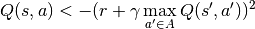and then we optimize using SGD just like a standard supervised learning problem.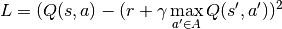#### DQN Results¶

DQN: Pong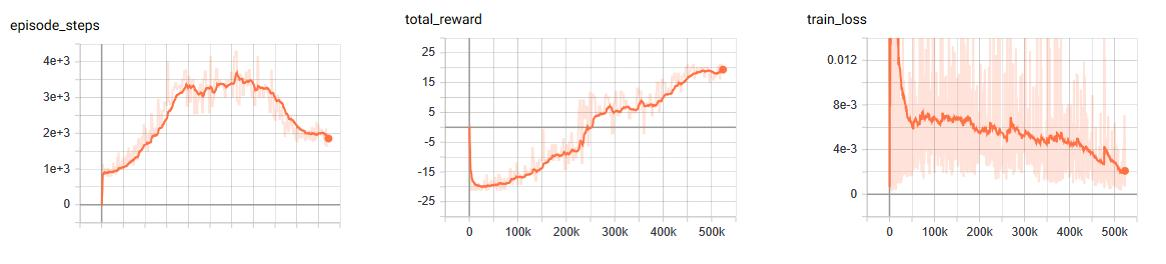Example:

from pl_bolts.models.rl import DQN
dqn = DQN("PongNoFrameskip-v4")
trainer = Trainer()
trainer.fit(dqn)

class pl_bolts.models.rl.dqn_model.DQN(env, eps_start=1.0, eps_end=0.02, eps_last_frame=150000, sync_rate=1000, gamma=0.99, learning_rate=0.0001, batch_size=32, replay_size=100000, warm_start_size=10000, avg_reward_len=100, min_episode_reward=- 21, seed=123, batches_per_epoch=1000, n_steps=1, **kwargs)[source]

Bases: pytorch_lightning.

Basic DQN Model

PyTorch Lightning implementation of DQN Paper authors: Volodymyr Mnih, Koray Kavukcuoglu, David Silver, Alex Graves, Ioannis Antonoglou, Daan Wierstra, Martin Riedmiller. Model implemented by:

• Donal Byrne <https://github.com/djbyrne>

Example

>>> from pl_bolts.models.rl.dqn_model import DQN
...
>>> model = DQN("PongNoFrameskip-v4")


Train:

trainer = Trainer()
trainer.fit(model)


Note

Currently only supports CPU and single GPU training with distributed_backend=dp

Parameters
static add_model_specific_args(arg_parser)[source]

Adds arguments for DQN model

Note

These params are fine tuned for Pong env.

Parameters

arg_parser (ArgumentParser) – parent parser

Return type

ArgumentParser

build_networks()[source]

Initializes the DQN train and target networks

Return type

None

configure_optimizers()[source]

Initialize Adam optimizer

Return type

List[Optimizer]

forward(x)[source]

Passes in a state x through the network and gets the q_values of each action as an output

Parameters

x (Tensor) – environment state

Return type

Tensor

Returns

q values

static make_environment(env_name, seed=None)[source]

Initialise gym environment

Parameters
Return type

object

Returns

gym environment

populate(warm_start)[source]

Populates the buffer with initial experience

Return type

None

run_n_episodes(env, n_epsiodes=1, epsilon=1.0)[source]

Carries out N episodes of the environment with the current agent

Parameters
Return type
test_dataloader()[source]

Get test loader

Return type

DataLoader

test_epoch_end(outputs)[source]

Log the avg of the test results

Return type
test_step(*args, **kwargs)[source]

Evaluate the agent for 10 episodes

Return type
train_batch()[source]

Contains the logic for generating a new batch of data to be passed to the DataLoader

Return type
Returns

yields a Experience tuple containing the state, action, reward, done and next_state.

train_dataloader()[source]

Get train loader

Return type

DataLoader

training_step(batch, _)[source]

Carries out a single step through the environment to update the replay buffer. Then calculates loss based on the minibatch recieved

Parameters
Return type

OrderedDict

Returns

Training loss and log metrics

### Double DQN¶

Double DQN model introduced in Deep Reinforcement Learning with Double Q-learning Paper authors: Hado van Hasselt, Arthur Guez, David Silver

Original implementation by: Donal Byrne

The original DQN tends to overestimate Q values during the Bellman update, leading to instability and is harmful to training. This is due to the max operation in the Bellman equation.

We are constantly taking the max of our agents estimates during our update. This may seem reasonable, if we could trust these estimates. However during the early stages of training, the estimates for these values will be off center and can lead to instability in training until our estimates become more reliable

The Double DQN fixes this overestimation by choosing actions for the next state using the main trained network but uses the values of these actions from the more stable target network. So we are still going to take the greedy action, but the value will be less “optimisitc” because it is chosen by the target network.

DQN expected return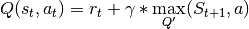Double DQN expected return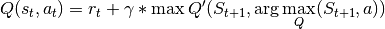#### Double DQN Results¶

Double DQN: Pong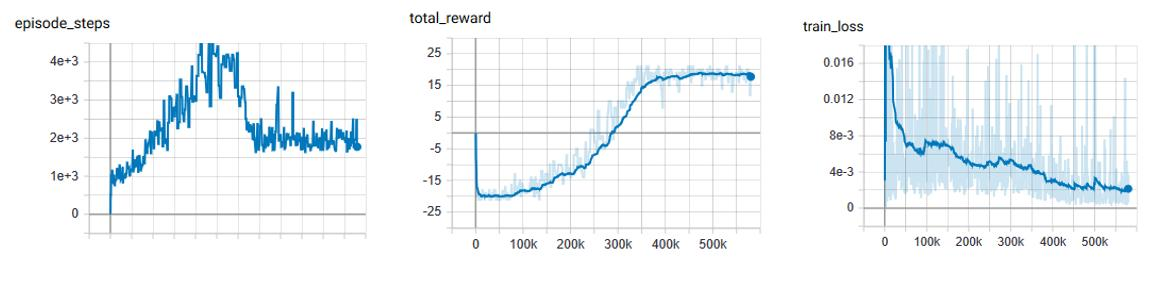DQN vs Double DQN: Pong

orange: DQN

blue: Double DQN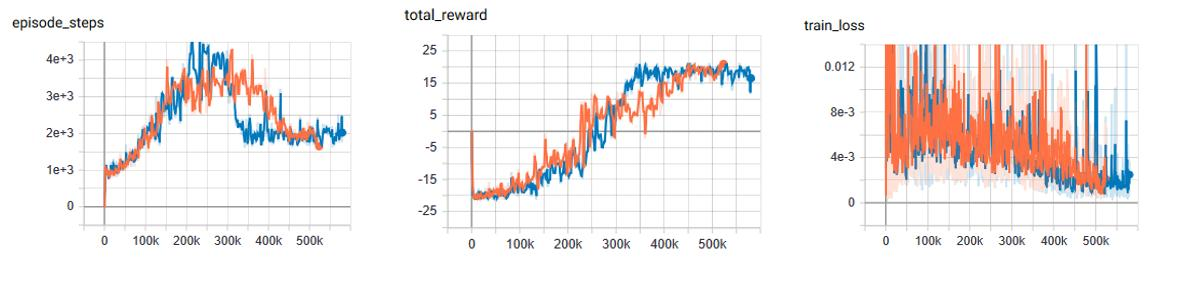Example:

from pl_bolts.models.rl import DoubleDQN
ddqn = DoubleDQN("PongNoFrameskip-v4")
trainer = Trainer()
trainer.fit(ddqn)

class pl_bolts.models.rl.double_dqn_model.DoubleDQN(env, eps_start=1.0, eps_end=0.02, eps_last_frame=150000, sync_rate=1000, gamma=0.99, learning_rate=0.0001, batch_size=32, replay_size=100000, warm_start_size=10000, avg_reward_len=100, min_episode_reward=- 21, seed=123, batches_per_epoch=1000, n_steps=1, **kwargs)[source]

Bases: pytorch_lightning.

Double Deep Q-network (DDQN) PyTorch Lightning implementation of Double DQN

Paper authors: Hado van Hasselt, Arthur Guez, David Silver

Model implemented by:

• Donal Byrne <https://github.com/djbyrne>

Example

>>> from pl_bolts.models.rl.double_dqn_model import DoubleDQN
...
>>> model = DoubleDQN("PongNoFrameskip-v4")


Train:

trainer = Trainer()
trainer.fit(model)


Note

Currently only supports CPU and single GPU training with distributed_backend=dp

Parameters
training_step(batch, _)[source]

Carries out a single step through the environment to update the replay buffer. Then calculates loss based on the minibatch recieved

Parameters
Return type

OrderedDict

Returns

Training loss and log metrics

### Dueling DQN¶

Dueling DQN model introduced in Dueling Network Architectures for Deep Reinforcement Learning Paper authors: Ziyu Wang, Tom Schaul, Matteo Hessel, Hado van Hasselt, Marc Lanctot, Nando de Freitas

Original implementation by: Donal Byrne

The Q value that we are trying to approximate can be divided into two parts, the value state V(s) and the ‘advantage’ of actions in that state A(s, a). Instead of having one full network estimate the entire Q value, Dueling DQN uses two estimator heads in order to separate the estimation of the two parts.

The value is the same as in value iteration. It is the discounted expected reward achieved from state s. Think of the value as the ‘base reward’ from being in state s.

The advantage tells us how much ‘extra’ reward we get from taking action a while in state s. The advantage bridges the gap between Q(s, a) and V(s) as Q(s, a) = V(s) + A(s, a).

In the paper Dueling Network Architectures for Deep Reinforcement Learning <https://arxiv.org/abs/1511.06581> the network uses two heads, one outputs the value state and the other outputs the advantage. This leads to better training stability, faster convergence and overall better results. The V head outputs a single scalar (the state value), while the advantage head outputs a tensor equal to the size of the action space, containing an advantage value for each action in state s.

Changing the network architecture is not enough, we also need to ensure that the advantage mean is 0. This is done by subtracting the mean advantage from the Q value. This essentially pulls the mean advantage to 0.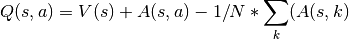#### Dueling DQN Benefits¶

• Ability to efficiently learn the state value function. In the dueling network, every Q update also updates the value stream, where as in DQN only the value of the chosen action is updated. This provides a better approximation of the values

• The differences between total Q values for a given state are quite small in relation to the magnitude of Q. The difference in the Q values between the best action and the second best action can be very small, while the average state value can be much larger. The differences in scale can introduce noise, which may lead to the greedy policy switching the priority of these actions. The seperate estimators for state value and advantage makes the Dueling DQN robust to this type of scenario

#### Dueling DQN Results¶

The results below a noticeable improvement from the original DQN network.

Dueling DQN baseline: Pong

Similar to the results of the DQN baseline, the agent has a period where the number of steps per episodes increase as it begins to hold its own against the heuristic oppoent, but then the steps per episode quickly begins to drop as it gets better and starts to beat its opponent faster and faster. There is a noticable point at step ~250k where the agent goes from losing to winning.

As you can see by the total rewards, the dueling network’s training progression is very stable and continues to trend upward until it finally plateus.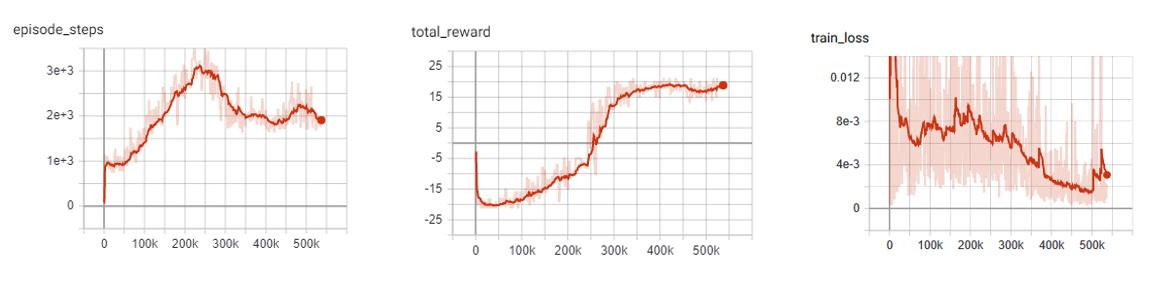DQN vs Dueling DQN: Pong

In comparison to the base DQN, we see that the Dueling network’s training is much more stable and is able to reach a score in the high teens faster than the DQN agent. Even though the Dueling network is more stable and out performs DQN early in training, by the end of training the two networks end up at the same point.

This could very well be due to the simplicity of the Pong environment.

• Orange: DQN

• Red: Dueling DQN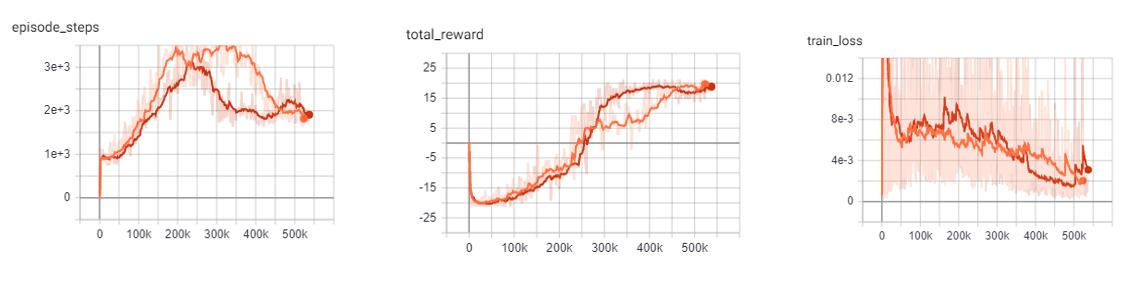Example:

from pl_bolts.models.rl import DuelingDQN
dueling_dqn = DuelingDQN("PongNoFrameskip-v4")
trainer = Trainer()
trainer.fit(dueling_dqn)

class pl_bolts.models.rl.dueling_dqn_model.DuelingDQN(env, eps_start=1.0, eps_end=0.02, eps_last_frame=150000, sync_rate=1000, gamma=0.99, learning_rate=0.0001, batch_size=32, replay_size=100000, warm_start_size=10000, avg_reward_len=100, min_episode_reward=- 21, seed=123, batches_per_epoch=1000, n_steps=1, **kwargs)[source]

Bases: pytorch_lightning.

PyTorch Lightning implementation of Dueling DQN

Paper authors: Ziyu Wang, Tom Schaul, Matteo Hessel, Hado van Hasselt, Marc Lanctot, Nando de Freitas

Model implemented by:

• Donal Byrne <https://github.com/djbyrne>

Example

>>> from pl_bolts.models.rl.dueling_dqn_model import DuelingDQN
...
>>> model = DuelingDQN("PongNoFrameskip-v4")


Train:

trainer = Trainer()
trainer.fit(model)


Note

Currently only supports CPU and single GPU training with distributed_backend=dp

Parameters
build_networks()[source]

Initializes the Dueling DQN train and target networks

Return type

None

### Noisy DQN¶

Noisy DQN model introduced in Noisy Networks for Exploration Paper authors: Meire Fortunato, Mohammad Gheshlaghi Azar, Bilal Piot, Jacob Menick, Ian Osband, Alex Graves, Vlad Mnih, Remi Munos, Demis Hassabis, Olivier Pietquin, Charles Blundell, Shane Legg

Original implementation by: Donal Byrne

Up until now the DQN agent uses a seperate exploration policy, generally epsilon-greedy where start and end values are set for its exploration. Noisy Networks For Exploration <https://arxiv.org/abs/1706.10295> introduces a new exploration strategy by adding noise parameters to the weights of the fully connect layers which get updated during backpropagation of the network. The noise parameters drive the exploration of the network instead of simply taking random actions more frequently at the start of training and less frequently towards the end. The of authors of propose two ways of doing this.

During the optimization step a new set of noisy parameters are sampled. During training the agent acts according to the fixed set of parameters. At the next optimization step, the parameters are updated with a new sample. This ensures the agent always acts based on the parameters that are drawn from the current noise distribution.

The authors propose two methods of injecting noise to the network.

1. Independent Gaussian Noise: This injects noise per weight. For each weight a random value is taken from the distribution. Noise parameters are stored inside the layer and are updated during backpropagation. The output of the layer is calculated as normal.

2. Factorized Gaussian Noise: This injects nosier per input/ouput. In order to minimize the number of random values this method stores two random vectors, one with the size of the input and the other with the size of the output. Using these two vectors, a random matrix is generated for the layer by calculating the outer products of the vector

#### Noisy DQN Benefits¶

• Improved exploration function. Instead of just performing completely random actions, we add decreasing amount of noise and uncertainty to our policy allowing to explore while still utilising its policy.

• The fact that this method is automatically tuned means that we do not have to tune hyper parameters for epsilon-greedy!

Note

For now I have just implemented the Independant Gaussian as it has been reported there isn’t much difference in results for these benchmark environments.

In order to update the basic DQN to a Noisy DQN we need to do the following

#### Noisy DQN Results¶

The results below improved stability and faster performance growth.

Noisy DQN baseline: Pong

Similar to the other improvements, the average score of the agent reaches positive numbers around the 250k mark and steadily increases till convergence.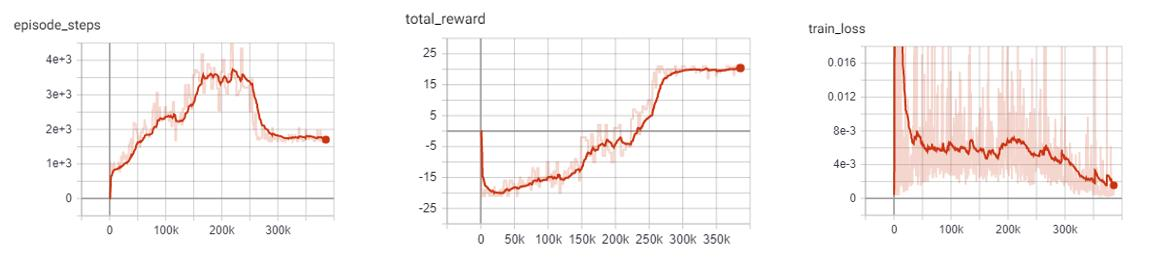DQN vs Noisy DQN: Pong

In comparison to the base DQN, the Noisy DQN is more stable and is able to converge on an optimal policy much faster than the original. It seems that the replacement of the epsilon-greedy strategy with network noise provides a better form of exploration.

• Orange: DQN

• Red: Noisy DQN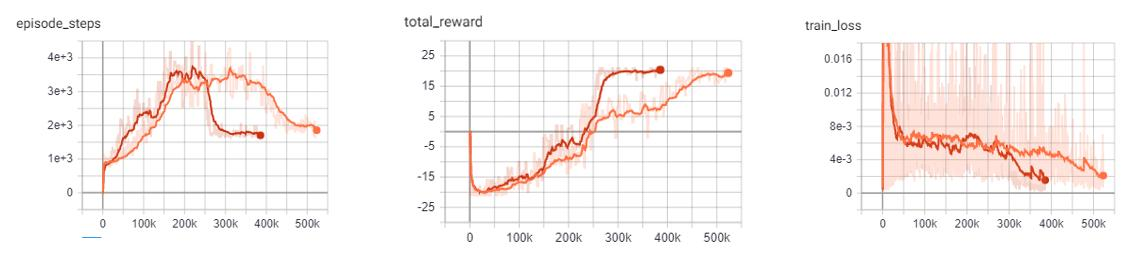Example:

from pl_bolts.models.rl import NoisyDQN
noisy_dqn = NoisyDQN("PongNoFrameskip-v4")
trainer = Trainer()
trainer.fit(noisy_dqn)

class pl_bolts.models.rl.noisy_dqn_model.NoisyDQN(env, eps_start=1.0, eps_end=0.02, eps_last_frame=150000, sync_rate=1000, gamma=0.99, learning_rate=0.0001, batch_size=32, replay_size=100000, warm_start_size=10000, avg_reward_len=100, min_episode_reward=- 21, seed=123, batches_per_epoch=1000, n_steps=1, **kwargs)[source]

Bases: pytorch_lightning.

PyTorch Lightning implementation of Noisy DQN

Paper authors: Meire Fortunato, Mohammad Gheshlaghi Azar, Bilal Piot, Jacob Menick, Ian Osband, Alex Graves, Vlad Mnih, Remi Munos, Demis Hassabis, Olivier Pietquin, Charles Blundell, Shane Legg

Model implemented by:

• Donal Byrne <https://github.com/djbyrne>

Example

>>> from pl_bolts.models.rl.noisy_dqn_model import NoisyDQN
...
>>> model = NoisyDQN("PongNoFrameskip-v4")


Train:

trainer = Trainer()
trainer.fit(model)


Note

Currently only supports CPU and single GPU training with distributed_backend=dp

Parameters
build_networks()[source]

Initializes the Noisy DQN train and target networks

Return type

None

on_train_start()[source]

Set the agents epsilon to 0 as the exploration comes from the network

Return type

None

train_batch()[source]

Contains the logic for generating a new batch of data to be passed to the DataLoader. This is the same function as the standard DQN except that we dont update epsilon as it is always 0. The exploration comes from the noisy network.

Return type
Returns

yields a Experience tuple containing the state, action, reward, done and next_state.

### N-Step DQN¶

N-Step DQN model introduced in Learning to Predict by the Methods of Temporal Differences Paper authors: Richard S. Sutton

Original implementation by: Donal Byrne

N Step DQN was introduced in Learning to Predict by the Methods of Temporal Differences. This method improves upon the original DQN by updating our Q values with the expected reward from multiple steps in the future as opposed to the expected reward from the immediate next state. When getting the Q values for a state action pair using a single step which looks like this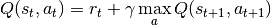but because the Q function is recursive we can continue to roll this out into multiple steps, looking at the expected return for each step into the future.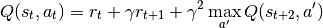The above example shows a 2-Step look ahead, but this could be rolled out to the end of the episode, which is just Monte Carlo learning. Although we could just do a monte carlo update and look forward to the end of the episode, it wouldn’t be a good idea. Every time we take another step into the future, we are basing our approximation off our current policy. For a large portion of training, our policy is going to be less than optimal. For example, at the start of training, our policy will be in a state of high exploration, and will be little better than random.

Note

For each rollout step you must scale the discount factor accordingly by the number of steps. As you can see from the equation above, the second gamma value is to the power of 2. If we rolled this out one step further, we would use gamma to the power of 3 and so.

So if we are aproximating future rewards off a bad policy, chances are those approximations are going to be pretty bad and every time we unroll our update equation, the worse it will get. The fact that we are using an off policy method like DQN with a large replay buffer will make this even worse, as there is a high chance that we will be training on experiences using an old policy that was worse than our current policy.

So we need to strike a balance between looking far enough ahead to improve the convergence of our agent, but not so far that are updates become unstable. In general, small values of 2-4 work best.

#### N-Step Benefits¶

• Multi-Step learning is capable of learning faster than typical 1 step learning methods.

• Note that this method introduces a new hyperparameter n. Although n=4 is generally a good starting point and provides good results across the board.

#### N-Step Results¶

As expected, the N-Step DQN converges much faster than the standard DQN, however it also adds more instability to the loss of the agent. This can be seen in the following experiments.

N-Step DQN: Pong

The N-Step DQN shows the greatest increase in performance with respect to the other DQN variations. After less than 150k steps the agent begins to consistently win games and achieves the top score after ~170K steps. This is reflected in the sharp peak of the total episode steps and of course, the total episode rewards.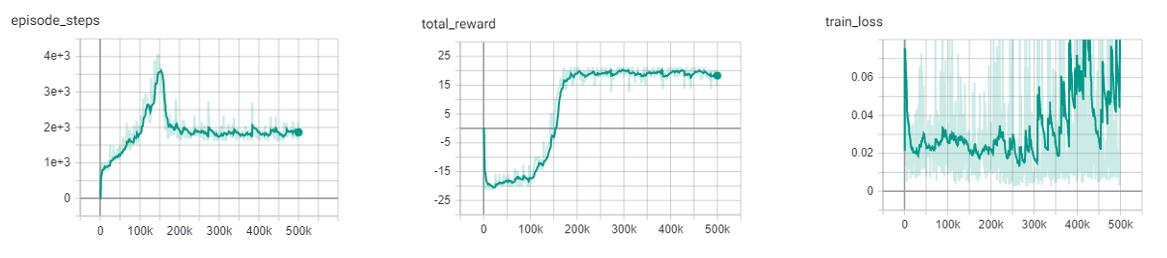DQN vs N-Step DQN: Pong

This improvement is shown in stark contrast to the base DQN, which only begins to win games after 250k steps and requires over twice as many steps (450k) as the N-Step agent to achieve the high score of 21. One important thing to notice is the large increase in the loss of the N-Step agent. This is expected as the agent is building its expected reward off approximations of the future states. The large the size of N, the greater the instability. Previous literature, listed below, shows the best results for the Pong environment with an N step between 3-5. For these experiments I opted with an N step of 4.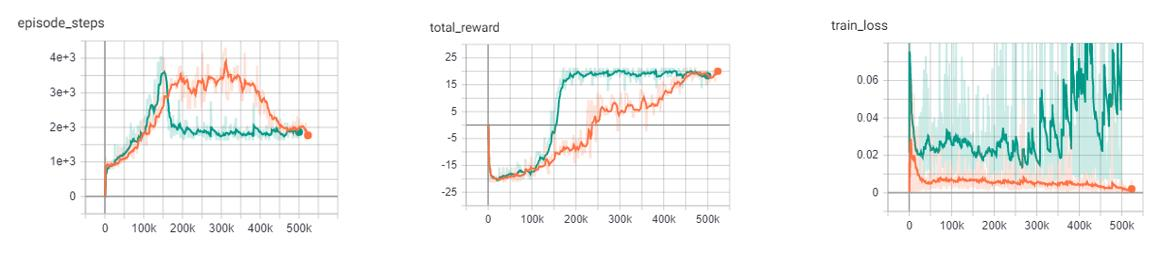Example:

from pl_bolts.models.rl import DQN
n_step_dqn = DQN("PongNoFrameskip-v4", n_steps=4)
trainer = Trainer()
trainer.fit(n_step_dqn)


### Prioritized Experience Replay DQN¶

Double DQN model introduced in Prioritized Experience Replay Paper authors: Tom Schaul, John Quan, Ioannis Antonoglou, David Silver

Original implementation by: Donal Byrne

The standard DQN uses a buffer to break up the correlation between experiences and uniform random samples for each batch. Instead of just randomly sampling from the buffer prioritized experience replay (PER) prioritizes these samples based on training loss. This concept was introduced in the paper Prioritized Experience Replay

Essentially we want to train more on the samples that sunrise the agent.

The priority of each sample is defined below where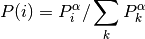where pi is the priority of the ith sample in the buffer and 𝛼 is the number that shows how much emphasis we give to the priority. If 𝛼 = 0 , our sampling will become uniform as in the classic DQN method. Larger values for 𝛼 put more stress on samples with higher priority

Its important that new samples are set to the highest priority so that they are sampled soon. This however introduces bias to new samples in our dataset. In order to compensate for this bias, the value of the weight is defined as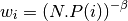Where beta is a hyper parameter between 0-1. When beta is 1 the bias is fully compensated. However authors noted that in practice it is better to start beta with a small value near 0 and slowly increase it to 1.

#### PER Benefits¶

• The benefits of this technique are that the agent sees more samples that it struggled with and gets more chances to improve upon it.

Memory Buffer

First step is to replace the standard experience replay buffer with the prioritized experience replay buffer. This is pretty large (100+ lines) so I wont go through it here. There are two buffers implemented. The first is a naive list based buffer found in memory.PERBuffer and the second is more efficient buffer using a Sum Tree datastructure.

The list based version is simpler, but has a sample complexity of O(N). The Sum Tree in comparison has a complexity of O(1) for sampling and O(logN) for updating priorities.

Update loss function

The next thing we do is to use the sample weights that we get from PER. Add the following code to the end of the loss function. This applies the weights of our sample to the batch loss. Then we return the mean loss and weighted loss for each datum, with the addition of a small epsilon value.

#### PER Results¶

The results below show improved stability and faster performance growth.

PER DQN: Pong

Similar to the other improvements, we see that PER improves the stability of the agents training and shows to converged on an optimal policy faster.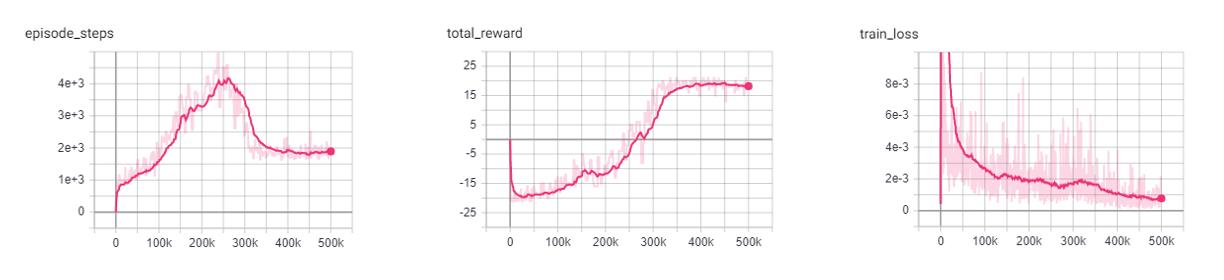DQN vs PER DQN: Pong

In comparison to the base DQN, the PER DQN does show improved stability and performance. As expected, the loss of the PER DQN is siginificantly lower. This is the main objective of PER by focusing on experiences with high loss.

It is important to note that loss is not the only metric we should be looking at. Although the agent may have very low loss during training, it may still perform poorly due to lack of exploration.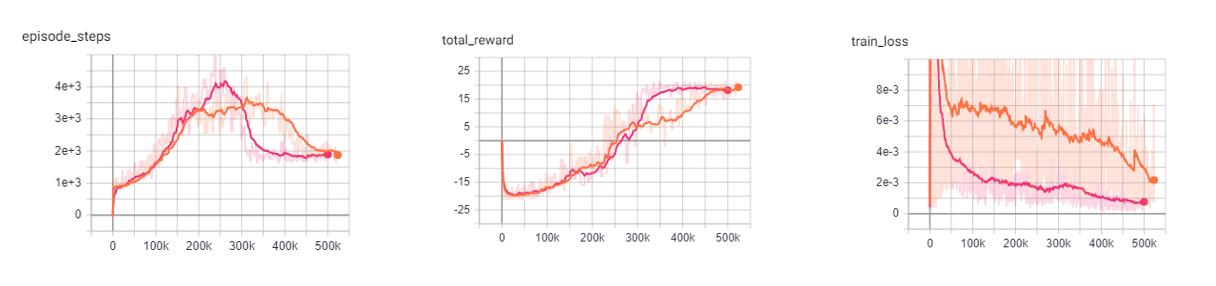• Orange: DQN

• Pink: PER DQN

Example:

from pl_bolts.models.rl import PERDQN
per_dqn = PERDQN("PongNoFrameskip-v4")
trainer = Trainer()
trainer.fit(per_dqn)

class pl_bolts.models.rl.per_dqn_model.PERDQN(env, eps_start=1.0, eps_end=0.02, eps_last_frame=150000, sync_rate=1000, gamma=0.99, learning_rate=0.0001, batch_size=32, replay_size=100000, warm_start_size=10000, avg_reward_len=100, min_episode_reward=- 21, seed=123, batches_per_epoch=1000, n_steps=1, **kwargs)[source]

Bases: pytorch_lightning.

PyTorch Lightning implementation of DQN With Prioritized Experience Replay

Paper authors: Tom Schaul, John Quan, Ioannis Antonoglou, David Silver

Model implemented by:

• Donal Byrne <https://github.com/djbyrne>

Example

>>> from pl_bolts.models.rl.per_dqn_model import PERDQN
...
>>> model = PERDQN("PongNoFrameskip-v4")


Train:

trainer = Trainer()
trainer.fit(model)


Note

Currently only supports CPU and single GPU training with distributed_backend=dp

Parameters
train_batch()[source]

Contains the logic for generating a new batch of data to be passed to the DataLoader

Return type
Returns

yields a Experience tuple containing the state, action, reward, done and next_state.

training_step(batch, _)[source]

Carries out a single step through the environment to update the replay buffer. Then calculates loss based on the minibatch recieved

Parameters
• batch – current mini batch of replay data

• _ – batch number, not used

Return type

OrderedDict

Returns

Training loss and log metrics

## Policy Gradient Models¶

The following models are based on Policy Gradients. Unlike the Q learning models shown before, Policy based models do not try and learn the specifc values of state or state action pairs. Instead it cuts out the middle man and directly learns the policy distribution. In Policy Gradient models we update our network parameters in the direction suggested by our policy gradient in order to find a policy that produces the highest results.

Policy Gradient Key Points:
• Outputs a distribution of actions instead of discrete Q values

• Optimizes the policy directly, instead of indirectly through the optimization of Q values

• The policy distribution of actions allows the model to handle more complex action spaces, such as continuous actions

• The policy distribution introduces stochasticity, providing natural exploration to the model

• The policy distribution provides a more stable update as a change in weights will only change the total distribution slightly, as opposed to changing weights based on the Q value of state S will change all Q values with similar states.

• Policy gradients tend to converge faste, however they are not as sample efficient and generally require more interactions with the environment.

### REINFORCE¶

REINFORCE model introduced in Policy Gradient Methods For Reinforcement Learning With Function Approximation Paper authors: Richard S. Sutton, David McAllester, Satinder Singh, Yishay Mansour

Original implementation by: Donal Byrne

REINFORCE is one of the simplest forms of the Policy Gradient method of RL. This method uses a Monte Carlo rollout, where its steps through entire episodes of the environment to build up trajectories computing the total rewards. The algorithm is as follows:

1. Initialize our network.

2. Play N full episodes saving the transitions through the environment.

3. For every step t in each episode k we calculate the discounted reward of the subsequent steps.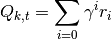1. Calculate the loss for all transitions.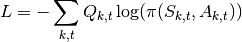1. Perform SGD on the loss and repeat.

What this loss function is saying is simply that we want to take the log probability of action A at state S given our policy (network output). This is then scaled by the discounted reward that we calculated in the previous step. We then take the negative of our sum. This is because the loss is minimized during SGD, but we want to maximize our policy.

Note

The current implementation does not actually wait for the batch episodes the complete every time as we pass in a fixed batch size. For the time being we simply use a large batch size to accomodate this. This approach still works well for simple tasks as it still manages to get an accurate Q value by using a large batch size, but it is not as accurate or completely correct. This will be updated in a later version.

#### REINFORCE Benefits¶

• Simple and straightforward

• Computationally more efficient for simple tasks such as Cartpole than the Value Based methods.

#### REINFORCE Results¶

Hyperparameters:

• Batch Size: 800

• Learning Rate: 0.01

• Episodes Per Batch: 4

• Gamma: 0.99

TODO: Add results graph

Example:

from pl_bolts.models.rl import Reinforce
reinforce = Reinforce("CartPole-v0")
trainer = Trainer()
trainer.fit(reinforce)

class pl_bolts.models.rl.reinforce_model.Reinforce(env, gamma=0.99, lr=0.01, batch_size=8, n_steps=10, avg_reward_len=100, entropy_beta=0.01, epoch_len=1000, num_batch_episodes=4, **kwargs)[source]

Bases: pytorch_lightning.

PyTorch Lightning implementation of REINFORCE Paper authors: Richard S. Sutton, David McAllester, Satinder Singh, Yishay Mansour Model implemented by:

• Donal Byrne <https://github.com/djbyrne>

Example

>>> from pl_bolts.models.rl.reinforce_model import Reinforce
...
>>> model = Reinforce("CartPole-v0")


Train:

trainer = Trainer()
trainer.fit(model)


Note

Currently only supports CPU and single GPU training with distributed_backend=dp

Parameters
static add_model_specific_args(arg_parser)[source]

Adds arguments for DQN model

Note

These params are fine tuned for Pong env.

Parameters

arg_parser – the current argument parser to add to

Return type

ArgumentParser

Returns

arg_parser with model specific cargs added

calc_qvals(rewards)[source]

Calculate the discounted rewards of all rewards in list

Parameters

rewards (List[float]) – list of rewards from latest batch

Return type
Returns

list of discounted rewards

configure_optimizers()[source]

Initialize Adam optimizer

Return type

List[Optimizer]

discount_rewards(experiences)[source]

Calculates the discounted reward over N experiences

Parameters

experiences (Tuple[Experience]) – Tuple of Experience

Return type

float

Returns

total discounted reward

forward(x)[source]

Passes in a state x through the network and gets the q_values of each action as an output

Parameters

x (Tensor) – environment state

Return type

Tensor

Returns

q values

get_device(batch)[source]

Retrieve device currently being used by minibatch

Return type

str

train_batch()[source]

Contains the logic for generating a new batch of data to be passed to the DataLoader

Yields

yields a tuple of Lists containing tensors for states, actions and rewards of the batch.

Return type
train_dataloader()[source]

Get train loader

Return type

DataLoader

training_step(batch, _)[source]

Carries out a single step through the environment to update the replay buffer. Then calculates loss based on the minibatch recieved

Parameters
Return type

OrderedDict

Returns

Training loss and log metrics

### Vanilla Policy Gradient¶

Vanilla Policy Gradient model introduced in Policy Gradient Methods For Reinforcement Learning With Function Approximation Paper authors: Richard S. Sutton, David McAllester, Satinder Singh, Yishay Mansour

Original implementation by: Donal Byrne

Vanilla Policy Gradient (VPG) expands upon the REINFORCE algorithm and improves some of its major issues. The major issue with REINFORCE is that it has high variance. This can be improved by subtracting a baseline value from the Q values. For this implementation we use the average reward as our baseline.

Although Policy Gradients are able to explore naturally due to the stochastic nature of the model, the agent can still frequently be stuck in a local optima. In order to improve this, VPG adds an entropy term to improve exploration.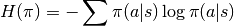To further control the amount of additional entropy in our model we scale the entropy term by a small beta value. The scaled entropy is then subtracted from the policy loss.

#### VPG Benefits¶

• Addition of the baseline reduces variance in the model

• Improved exploration due to entropy bonus

#### VPG Results¶

Hyperparameters:

• Batch Size: 8

• Learning Rate: 0.001

• N Steps: 10

• N environments: 4

• Entropy Beta: 0.01

• Gamma: 0.99

Example:

from pl_bolts.models.rl import VanillaPolicyGradient
vpg = VanillaPolicyGradient("CartPole-v0")
trainer = Trainer()
trainer.fit(vpg)

class pl_bolts.models.rl.vanilla_policy_gradient_model.VanillaPolicyGradient(env, gamma=0.99, lr=0.01, batch_size=8, n_steps=10, avg_reward_len=100, entropy_beta=0.01, epoch_len=1000, **kwargs)[source]

Bases: pytorch_lightning.

PyTorch Lightning implementation of Vanilla Policy Gradient

Paper authors: Richard S. Sutton, David McAllester, Satinder Singh, Yishay Mansour

Model implemented by:

• Donal Byrne <https://github.com/djbyrne>

Example

>>> from pl_bolts.models.rl.vanilla_policy_gradient_model import VanillaPolicyGradient
...
>>> model = VanillaPolicyGradient("CartPole-v0")


Train:

trainer = Trainer()
trainer.fit(model)


Note

Currently only supports CPU and single GPU training with distributed_backend=dp

Parameters
static add_model_specific_args(arg_parser)[source]

Adds arguments for DQN model

Note

These params are fine tuned for Pong env.

Parameters

arg_parser – the current argument parser to add to

Return type

ArgumentParser

Returns

arg_parser with model specific cargs added

compute_returns(rewards)[source]

Calculate the discounted rewards of the batched rewards

Parameters

rewards – list of batched rewards

Returns

list of discounted rewards

configure_optimizers()[source]

Initialize Adam optimizer

Return type

List[Optimizer]

forward(x)[source]

Passes in a state x through the network and gets the q_values of each action as an output

Parameters

x (Tensor) – environment state

Return type

Tensor

Returns

q values

get_device(batch)[source]

Retrieve device currently being used by minibatch

Return type

str

loss(states, actions, scaled_rewards)[source]

Calculates the loss for VPG

Parameters
• states – batched states

• actions – batch actions

• scaled_rewards – batche Q values

Return type

Tensor

Returns

loss for the current batch

train_batch()[source]

Contains the logic for generating a new batch of data to be passed to the DataLoader

Return type
Returns

yields a tuple of Lists containing tensors for states, actions and rewards of the batch.

train_dataloader()[source]

Get train loader

Return type

DataLoader

training_step(batch, _)[source]

Carries out a single step through the environment to update the replay buffer. Then calculates loss based on the minibatch recieved

Parameters
Return type

OrderedDict

Returns

Training loss and log metrics

© Copyright Copyright (c) 2020-2021, PyTorchLightning et al.. Revision 9044ed14.

Built with Sphinx using a theme provided by Read the Docs.
Read the Docs v: latest
Versions
latest
stable
0.3.2
0.3.1
0.3.0
0.2.5
0.2.4
0.2.3
0.2.2
0.2.1
0.2.0
0.1.1
docs-build-rtd
0.1.0
Downloads
pdf
html
On Read the Docs
Project Home
Builds

Free document hosting provided by Read the Docs.